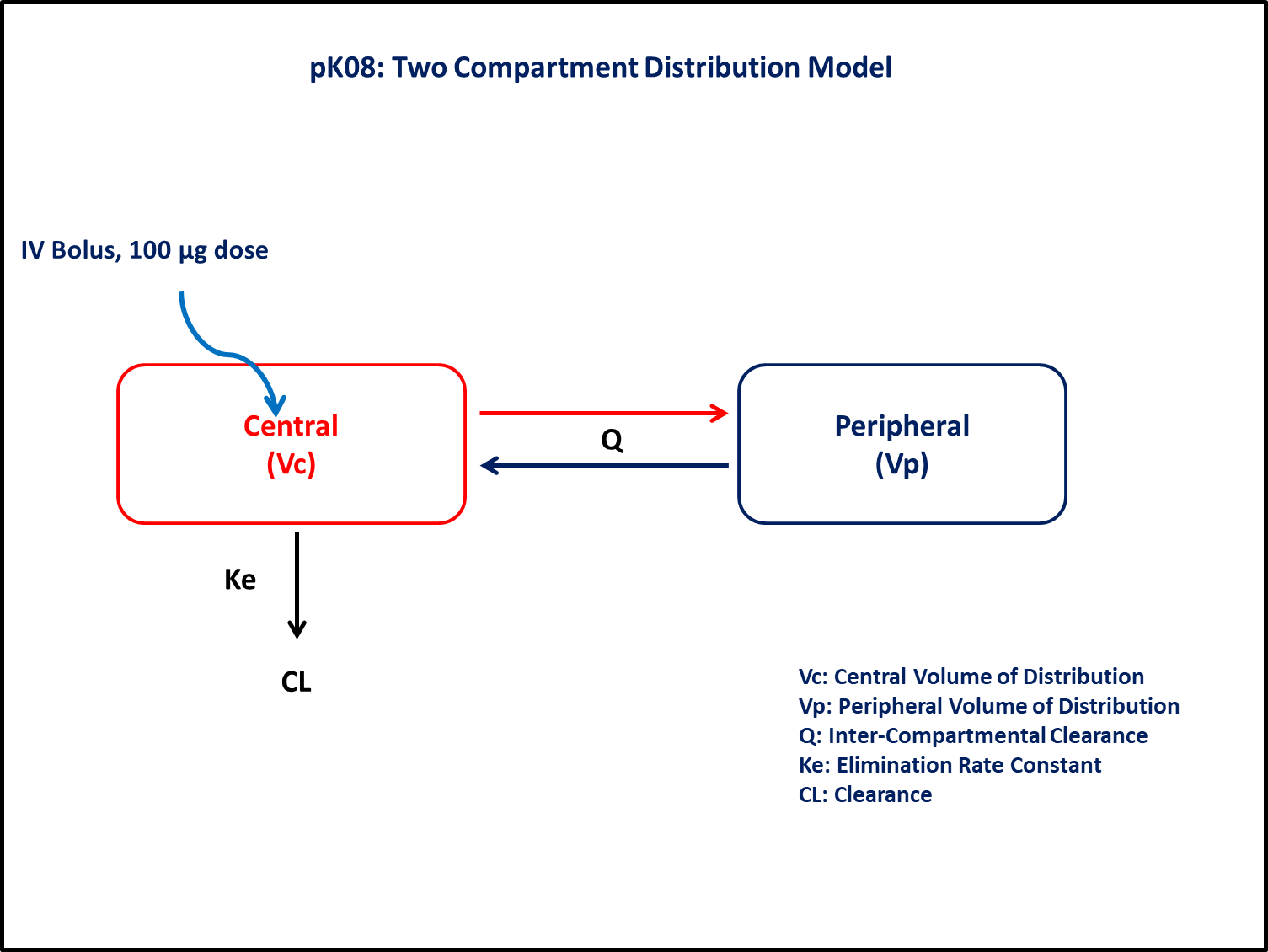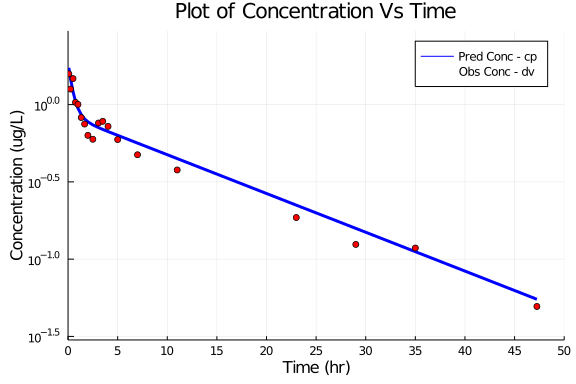# Exercise PK08 - Two-Compartment Distribution Models (Part 5 - Using Diffferential Equations)

### Background

• Structural model - Two compartment linear elimination with first order elimination

• Route of administration - IV bolus,

• Dosage Regimen - 100 μg IV or 0.1 mg IV

• Number of Subjects - 1### Learning Outcome

This exercise explains about the two compartment modelling with analyzing the parameters to be taken and simulate it for single subject given single IV bolus dose.

### Objectives

To build two compartment model and to simulate the model for a single subject given a single IV bolus dose.

### Libraries

call the "necessary" libraries to get started.

using Pumas
using Plots
using CSV
using StatsPlots
using Random


### Model

To build the two compartment model for a single subject given IV dose of 100micrograms and concentration-time graph was plotted for 50 hours specified in terms of differentiall equations considering plasma clearance(CL), the volume of central compartment(Vc),inter-compartmental distribution(Q) and volume of peripheral compartment(Vp) as initial parameters for simulation of plot.

pk_08_05        = @model begin
@param begin
tvcl        ∈ RealDomain(lower=0)
tvvc        ∈ RealDomain(lower=0)
tvq         ∈ RealDomain(lower=0)
tvvp        ∈ RealDomain(lower=0)
Ω           ∈ PDiagDomain(4)
σ²_prop     ∈ RealDomain(lower=0)
end

@random begin
η           ~ MvNormal(Ω)
end

@pre begin
Cl          = tvcl * exp(η)
Vc          = tvvc * exp(η)
Vp          = tvvp * exp(η)
Q           = tvq * exp(η)
end

@dynamics begin
Central'    = -(Cl/Vc)*Central - (Q/Vc)*Central + (Q/Vp)*Peripheral
Peripheral' =  (Q/Vc)*Central  - (Q/Vp)*Peripheral
end

@derived begin
cp          = @. Central/Vc
dv          ~ @. Normal(cp, sqrt(cp^2*σ²_prop))
end
end

PumasModel
Parameters: tvcl, tvvc, tvq, tvvp, Ω, σ²_prop
Random effects: η
Covariates:
Dynamical variables: Central, Peripheral
Derived: cp, dv
Observed: cp, dv


### Parameters

• CL - Clearance(L/hr),

• Vc - Volume of Central Compartment(L),

• Vp - Volume of Peripheral Compartment(L),

• Q - Inter-departmental clearance(L/hr),

• Ω - Between Subject Variability,

• σ - Residual error

param  = (tvcl    = 6.6,
tvvc    = 53.09,
tvvp    = 57.22,
tvq     = 51.5,
Ω       = Diagonal([0.0,0.0,0.0,0.0]),
σ²_prop = 0.047)

(tvcl = 6.6, tvvc = 53.09, tvvp = 57.22, tvq = 51.5, Ω = [0.0 0.0 0.0 0.0;
0.0 0.0 0.0 0.0; 0.0 0.0 0.0 0.0; 0.0 0.0 0.0 0.0], σ²_prop = 0.047)


### Dosage Regimen

Dosage Regimen - 100 μg or 0.1mg of IV bolus was given to the single subject.

ev1   = DosageRegimen(100,time=0,cmt=1,evid=1,addl=0,ii=0)
sub1  = Subject(id=1,events=ev1)

Subject
ID: 1
Events: 1


### Simulation

To simultate the plasma concentration with given observation time-points for single subject.

Random.seed!(123)
sim_s1 = simobs(pk_08_05,sub1,param,
obstimes=[0.08,0.25,0.5,0.75,1,1.33,1.67,2,2.5,3.07,3.5,4.03,5,7,11,23,29,35,47.25]);


### Dataframe and Plot

Generate a dataframe from the simulation and use it for plotting.

df1 = DataFrame(sim_s1)

# Plot the Plasma Concentration vs Time

@df df1 plot(:time,:cp, yaxis=:log,
label="Pred Conc - cp", xlabel= "Time (hr)", ylabel="Concentration (ug/L)", title="Plot of Concentration Vs Time",
xticks=[0,5,10,15,20,25,30,35,40,45,50],xlims=(0,50), ylims=(0.03,3),
color=[:blue], linestyle=[:solid], linewidth=3)
@df df1 scatter!(:time,:dv,
label="Obs Conc - dv", color=[:red], markershape=[:circle])